# Balanced Comparison With Multiple Concepts

 The Balanced Comparison feature is currently set up to support studies with two concepts. Here's a strategy for how you can set up Balanced Comparison to test more than two concepts

Balanced Comparison is available on the following subscriptions:

 Flex plan Seat-based plan Essentials Startup Advanced ✓ Professional ✓ Ultimate ✓ Premium ✓

Balanced Comparison, Three Concepts

Balanced Comparison, Four Concepts

Balanced Comparison, Five Concepts

Balanced Comparison, Six Concepts

Related Content

# Balanced Comparison, Three Concepts

Here is how you set up a Balanced comparison with three concepts:

3 Concept Setup v1a

1. Set up a website study

First Study (3 Concept Setup v1a)3 Concept Setup v1b

1. Create a copy of the setup v1a
2. Move the Concept 3 Task group above the Balanced Comparison

Second Study (3 Concept Setup v1b)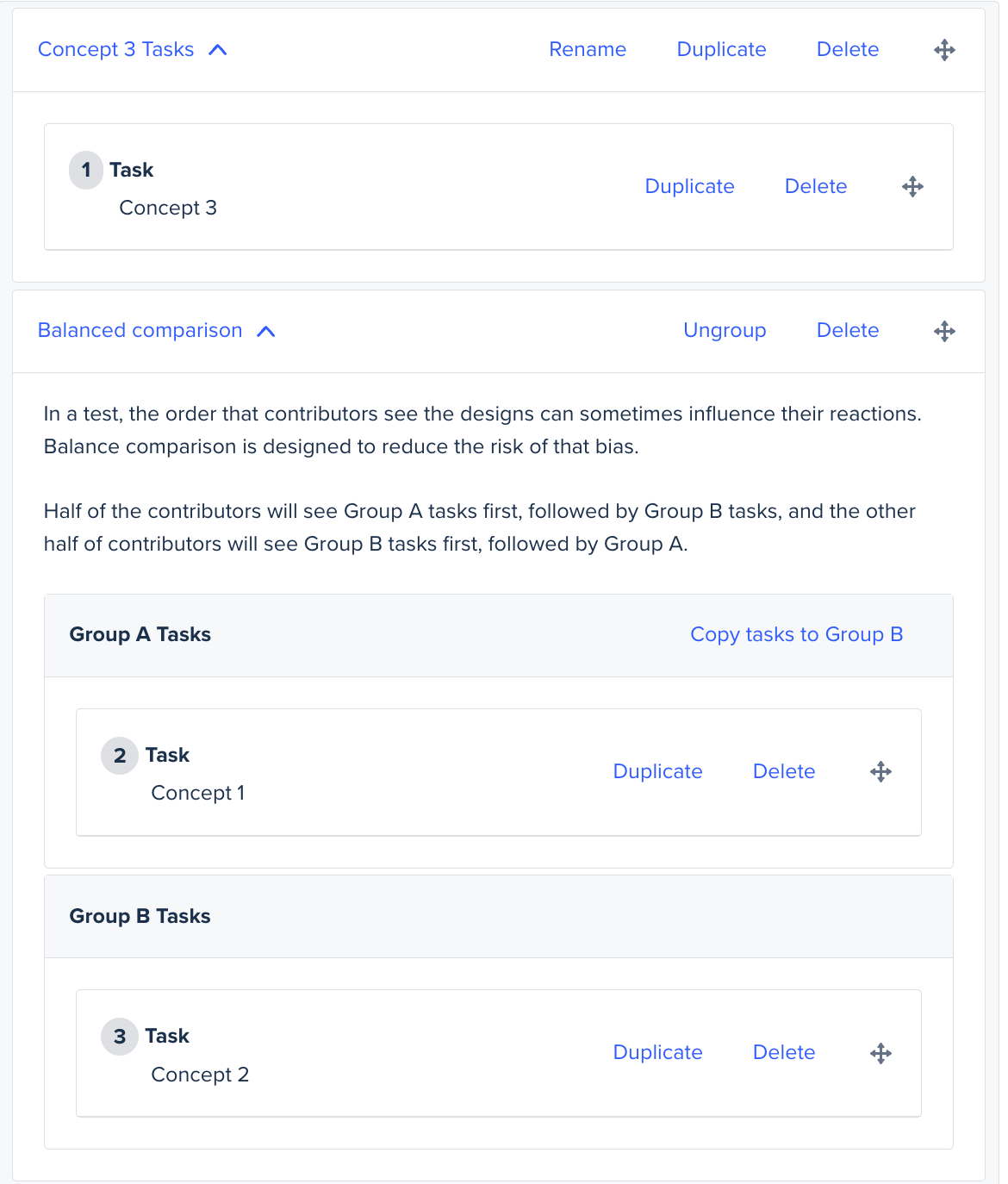Note: The contributors should be balanced across the studies.

Explanation:
Two studies will need to be run for 3 concepts (let's call them concept 1/2/3). The first study (3 Concept Setup v1a) will have concept 1 in Group A Tasks, concept 2 in Group B Tasks, and concept 3 as a Custom task group after the Balanced Comparison.

The second study (3 Concept Setup v1b) will have concept 3 preceding the Balanced Comparison in a Custom task group, with concepts 1 and 2 remaining in Group A and B Tasks, respectively.

This is how contributors will be shown your concepts:

Study 1 - Half the contributors will see concepts 1, 2, 3, and the other half will see concepts 2, 1, 3.

Study 2 - Half the contributors will be shown in concepts 3, 1, 2, and the other half in concepts 3, 2, 1.

## How to balance contributors across two studies with 3 concepts:

In order to get the best results, we suggest balancing the number of contributors between the 3 concepts, so an equal number of contributors will see each concept first. This should remove any bias. Since Study 2 will always show the 3rd concept first, it will only need half as many contributors as Study 1.

Example: If you want results from 6 contributors in total (2 for each concept first), then please launch Study 1 with 4 contributors and Study 2 with 2 contributors. If you want results from 12 contributors (4 contributors see each concept first), we suggest having 8 contributors take Study 1, and 4 contributors take Study 2. This way, the same number of contributors sees each concept first.

6 Contributors (2 for each concept):

Study 1 = 4 contributors

Study 2 = 2 contributors

12 Contributors (4 for each concept):

Study 1 = 8 contributors

Study 2 = 4 contributors

## Balanced Comparison, Four Concepts

Here is how you set up a Balanced comparison with four concepts:

4 Concept Setup v1a

1. Set up a website study

First Study (4 Concept Setup v1a)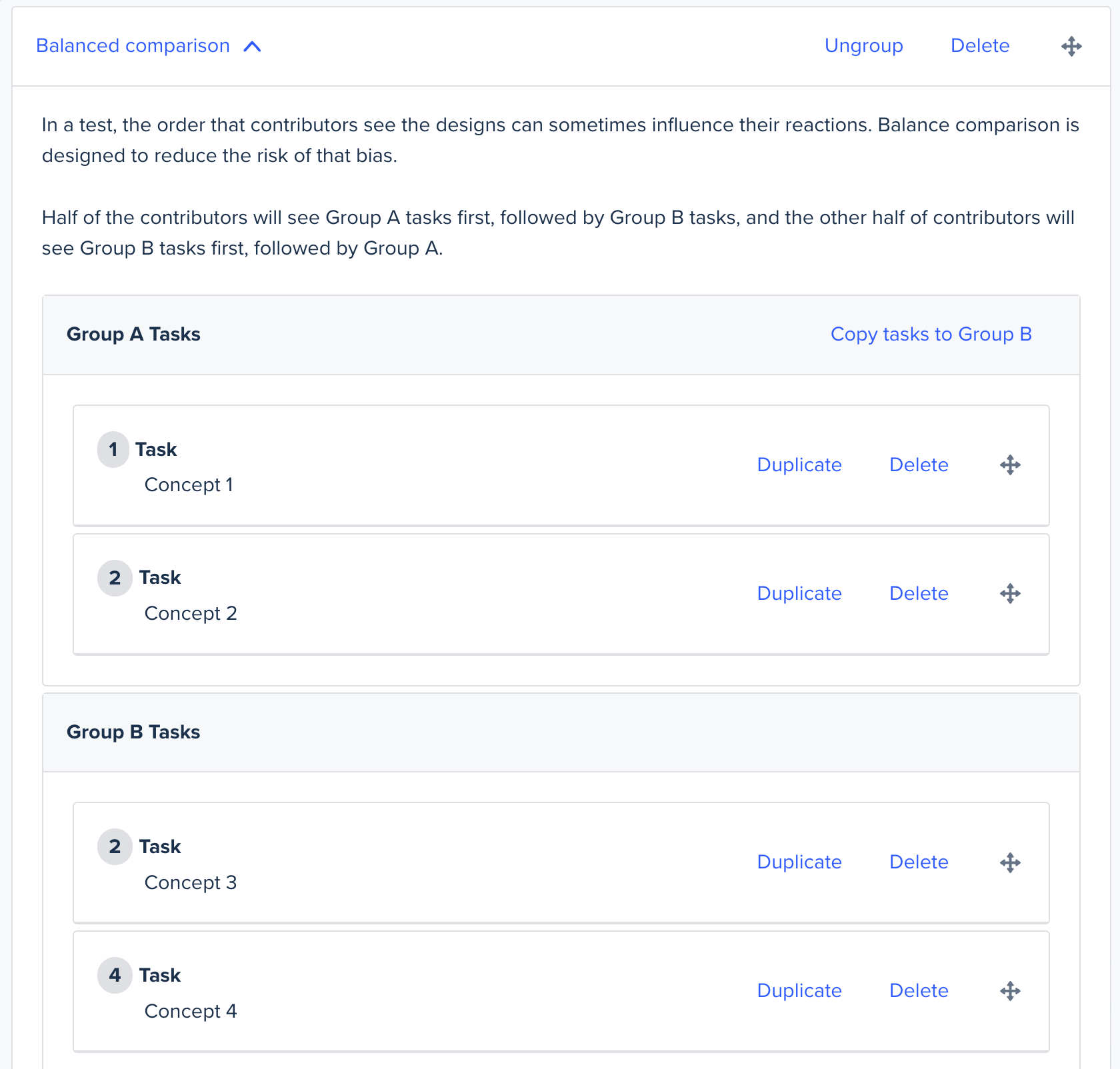4 Concept Setup v1b

1. Create a copy of the setup v1a
2. Switch the tasks for the 1st and 2nd concept around, so the 2nd concept is first
3. Switch the tasks for the 3rd and 4th concept around, so the 4th concept is first

Second Study (4 Concept Setup v1b)Note: An equal number of contributors should take each study.

Explanation:
For 4 concepts (let's call them concept 1/2/3/4), two studies will need to be run. The first study will have concept 1 and 2 in Group A Tasks, concept 3 and 4 in Group B Tasks.

The second study will have concept 2 and 1 in Group A Tasks and concept 4 and 3 in Group B Tasks.

This is how contributors will be shown your concepts:

Study 1 - Half the contributors will see 1, 2, 3, 4, and the other half will see 3, 4, 1, 2.

Study 2 - Half the contributors will see 2, 1, 4, 3, and the other half will see 4, 3, 2, 1.

Note: An equal number of contributors should take each study.

## Balanced Comparison Five Concepts

Here is how you set up a Balanced comparison with five concepts:

5 Concept Setup v1a

1. Set up a website study

First Study (5 Concept Setup v1a)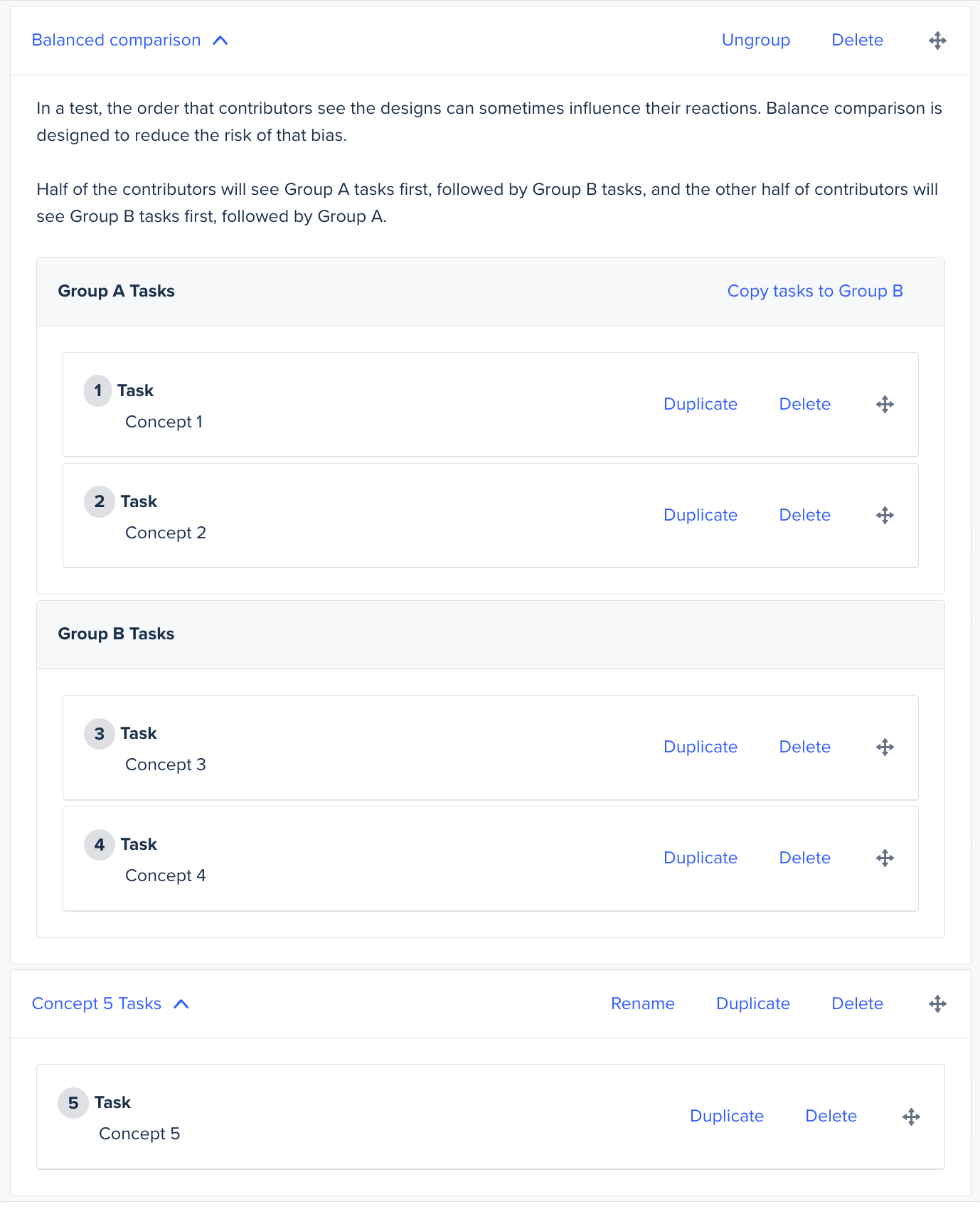5 Concept Setup v1b

1. Create a copy of the setup v1a
2. Switch the tasks for the 1st and 2nd concept around, so the 2nd concept is first
3. Switch the tasks for the 3rd and 4th concept around, so the 4th concept is first

Second Study (5 Concept Setup v1b)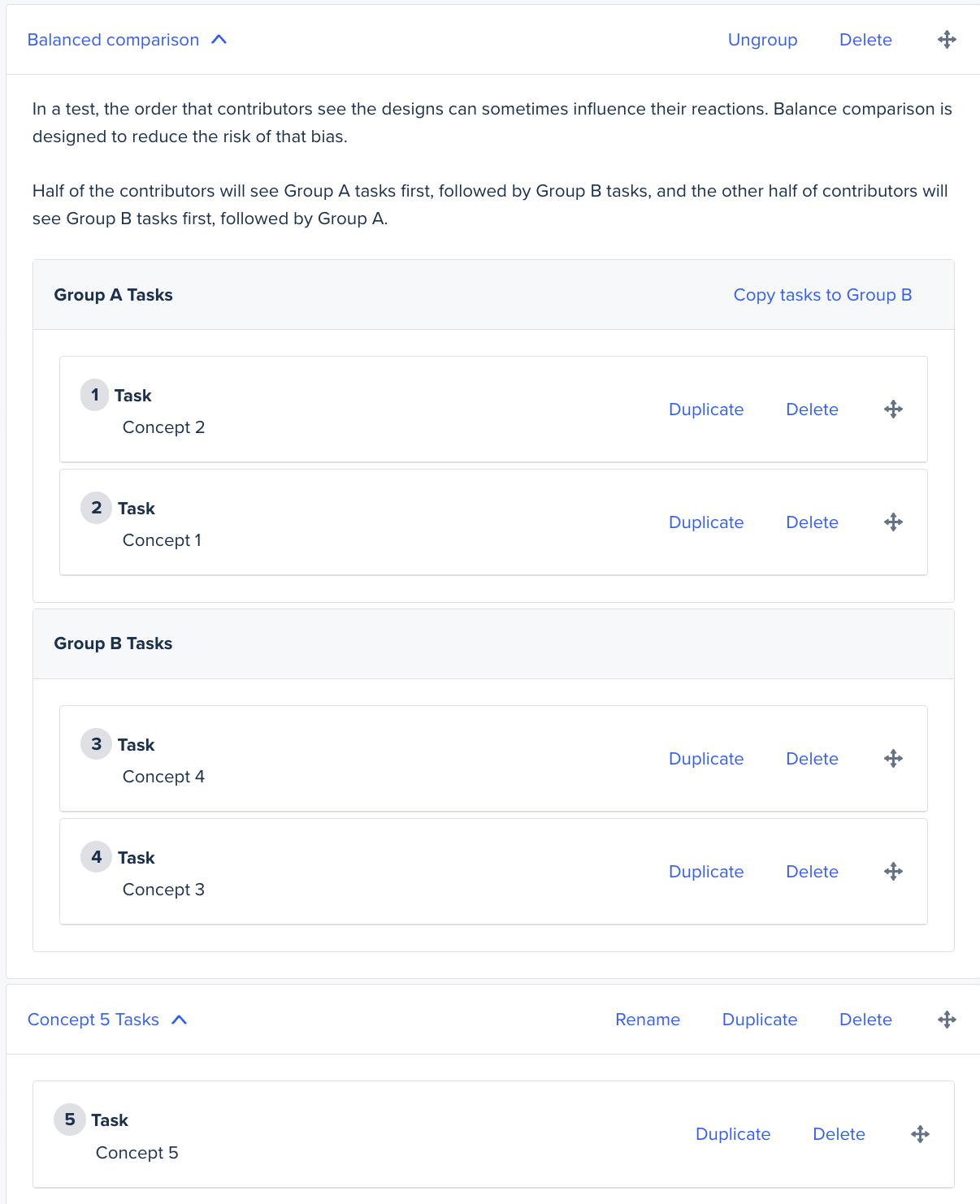5 Concept Setup v1c

1. Create a copy of the setup v1a
2.  Move the Concept 5 Task group above the Balanced Comparison

Third Study (5 Concept v1c)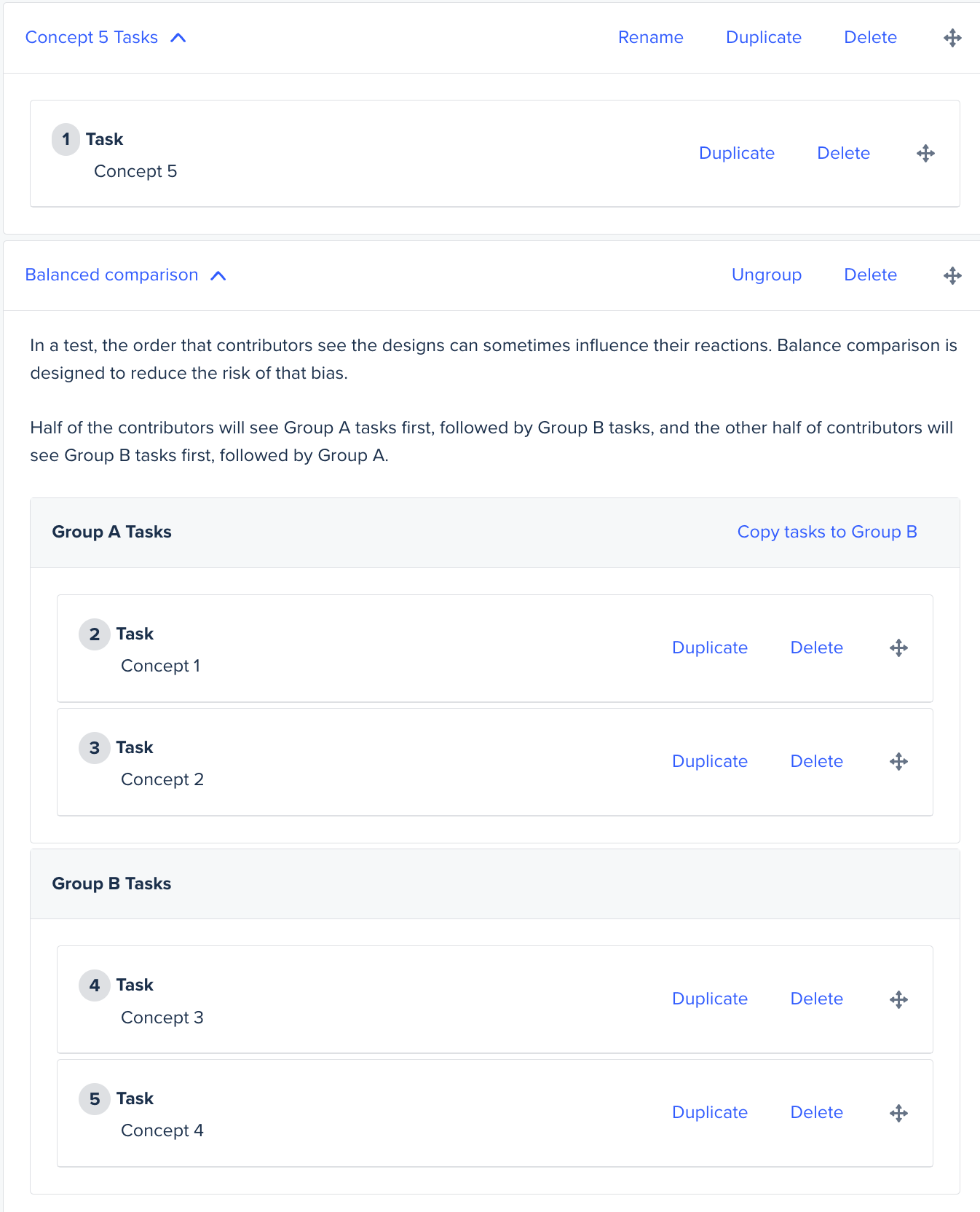Note: The contributors should be balanced across the studies.

Explanation:
For 5 concepts (let's call them concept 1/2/3/4/5), three studies will need to be run. The first study will have concept 1 and 2 in Group A Tasks, concept 3 and 4 in Group B Tasks, and concept 5 in the subsequent custom task group.

The second study will have concept 2 and 1 in Group A Tasks, option 4 and 3 in Group B Tasks, and option 5 in the subsequent custom task groups.

The third study will have concept 5 in the custom task group preceding the other 4 concepts in Groups A and B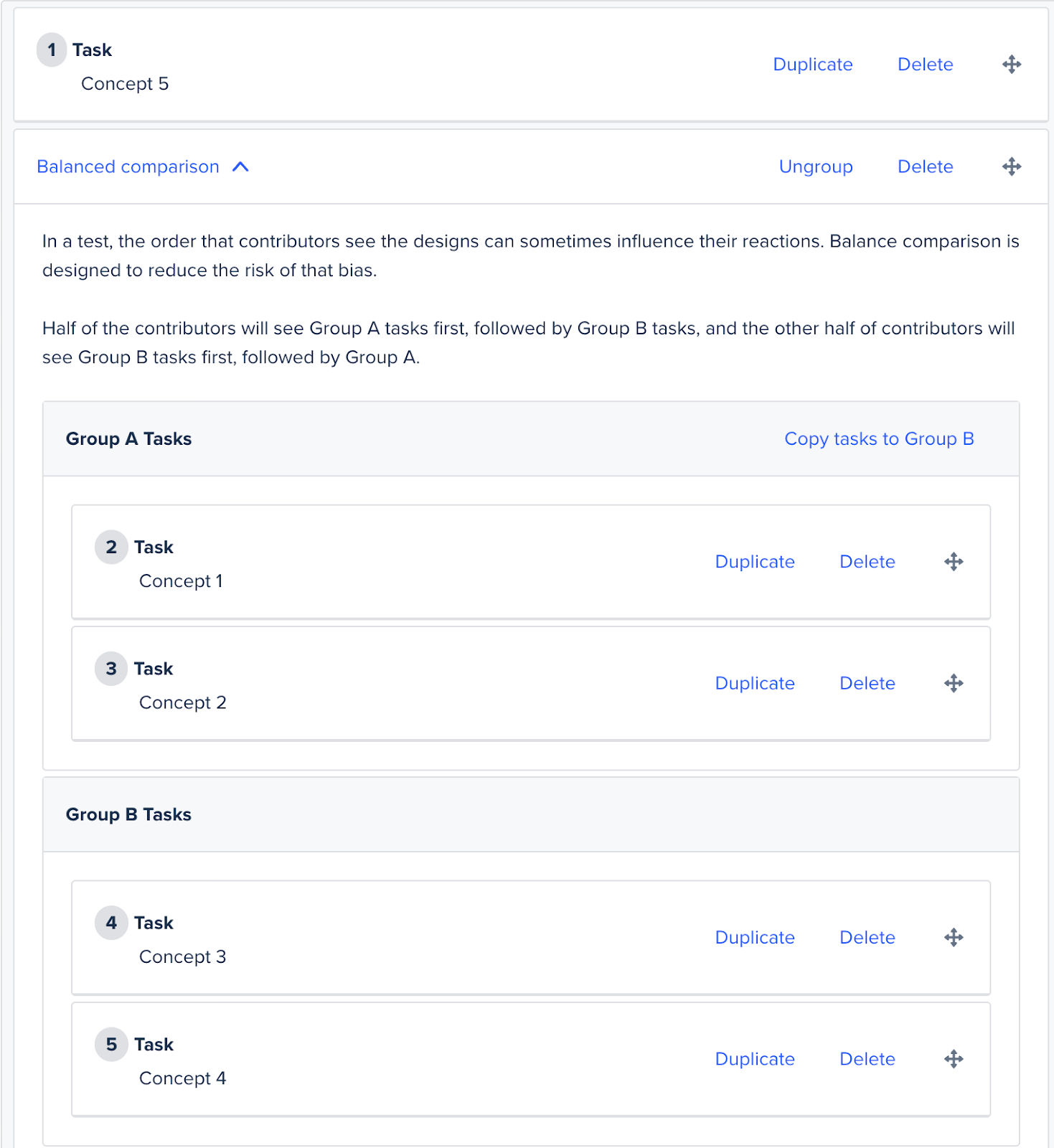This is how contributors will be shown your concepts:

Study 1 - Half the contributors will see 1, 2, 3, 4, 5, and the other half will see 3, 4, 1, 2, 5.

Study 2 - Half the contributors will see 2, 1, 4, 3, 5, and the other half will see 4, 3, 2, 1, 5.

Study 3 - Half the contributors will see 5, 1, 2, 3, 4, and the other half will see 5, 3, 4, 1, 2.

## How to balance contributors across three studies with 5 concepts:

In order to get the best results, we suggest balancing the number of contributors between the 5 concepts, so an equal number of contributors will see each concept first and this should remove any bias. Since the third study always shows the 5th concept first, then it only needs half as many contributors of the first and second study.

If you want results from 10 contributors total (2 for each concept), then please have study 1 and 2 have 4 contributors each, while study 3 should have 2 contributors. If you want results from 20 (4 for each concept) contributors total, then please have 8 contributors take study 1 and 2, and 4 take study 3. This way, the same number of contributors sees each concept first.

10 Participants (2 for each concept):

Study 1 = 4 contributors

Study 2 = 4 contributors

Study 3 = 2 contributors

20 Participants (4 for each concept):

Study 1 = 8 contributors

Study 2 = 8 contributors

Study 3 = 4 contributors

## Balanced Comparison, Six Concepts

Here is how you set up a Balanced comparison with six concepts:

6 Concept Setup v1a

1. Set up a website study
3. Add the 1st, 2nd and 3rd concept in Group A Tasks
4. Add the 4th, 5th and 6th concept in Group B Tasks

First Study (6 Concept Setup v1a)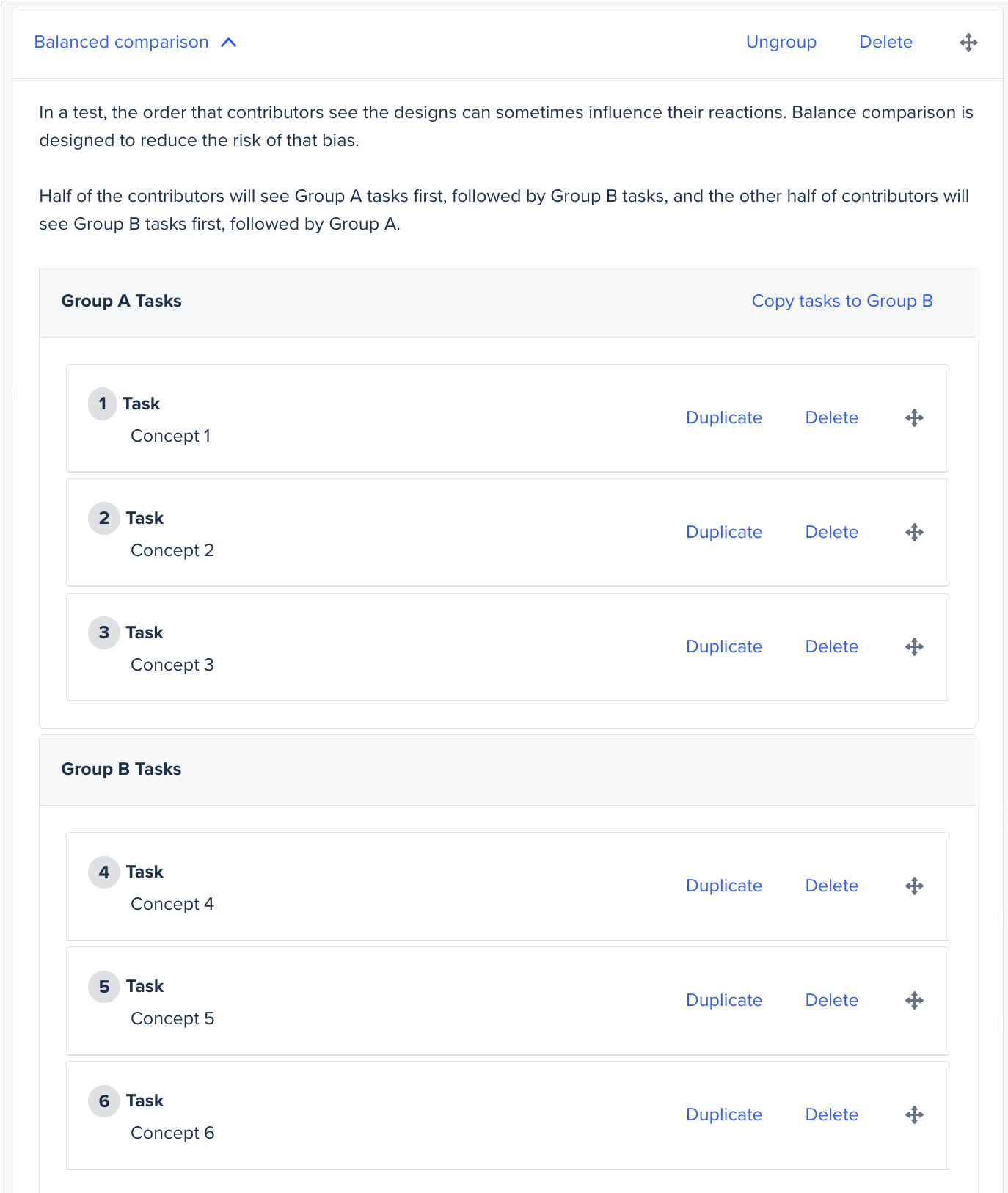6 Concept Setup v1b

1. Create a copy of the setup v1a
2. Switch the tasks for the 1st concept to the bottom of Group A Tasks so that the order is concepts 2 3 1.
3. Switch the tasks for the 4th concept to the bottom of Group B Tasks so that the order is concepts 5 6 4.

Second Study (6 Concept Setup v1b)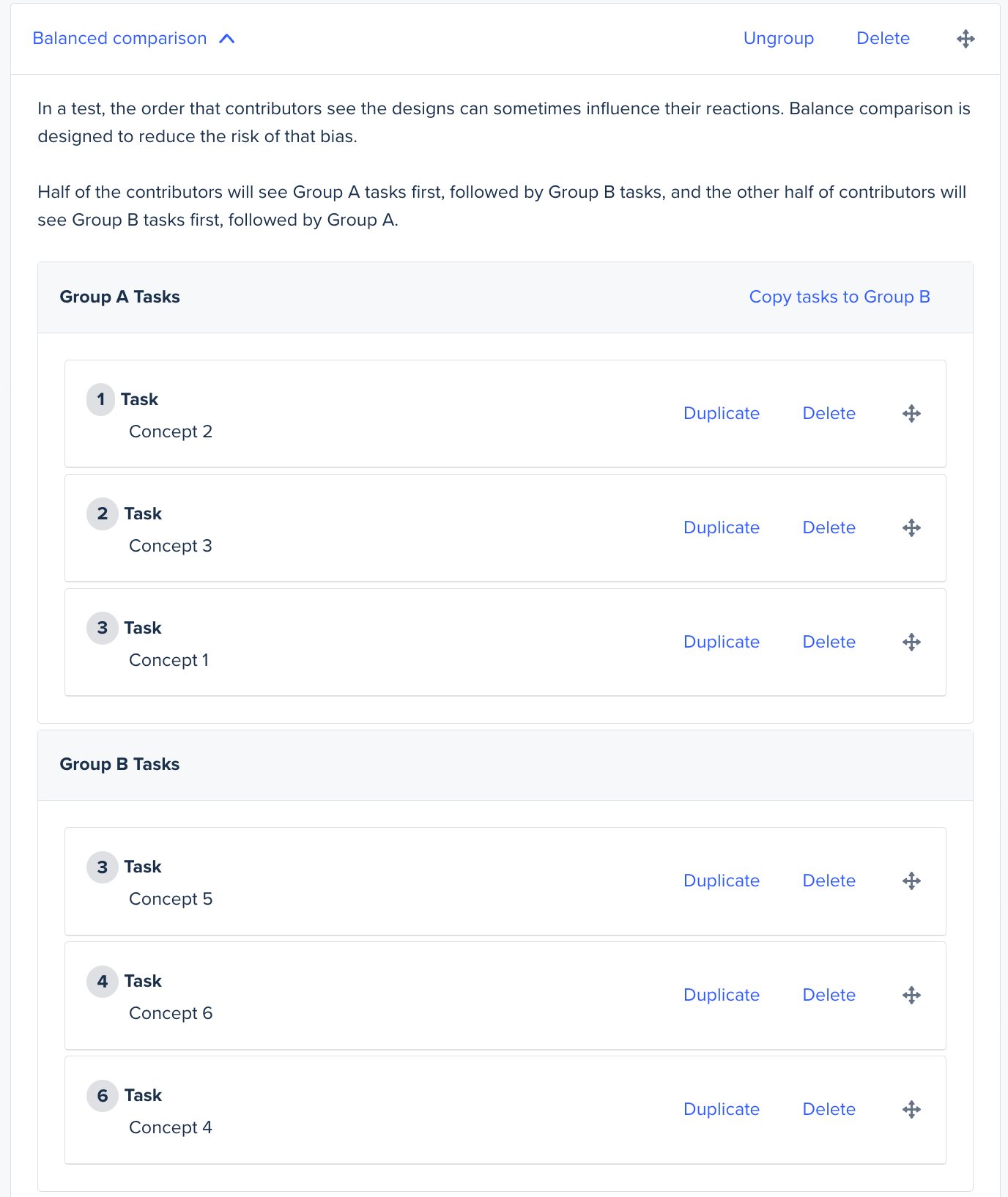6 Concept Setup v1c

1. Create a copy of the setup v1b
2. Switch the tasks for the 2nd concept to the bottom of Group A Tasks, so that the order is concepts 3 1 2.
3. Switch the tasks for the 5th concept to the bottom of Group B Tasks, so that the order is concepts 6 4 5.

Third Study (6 Concept Setup v1c)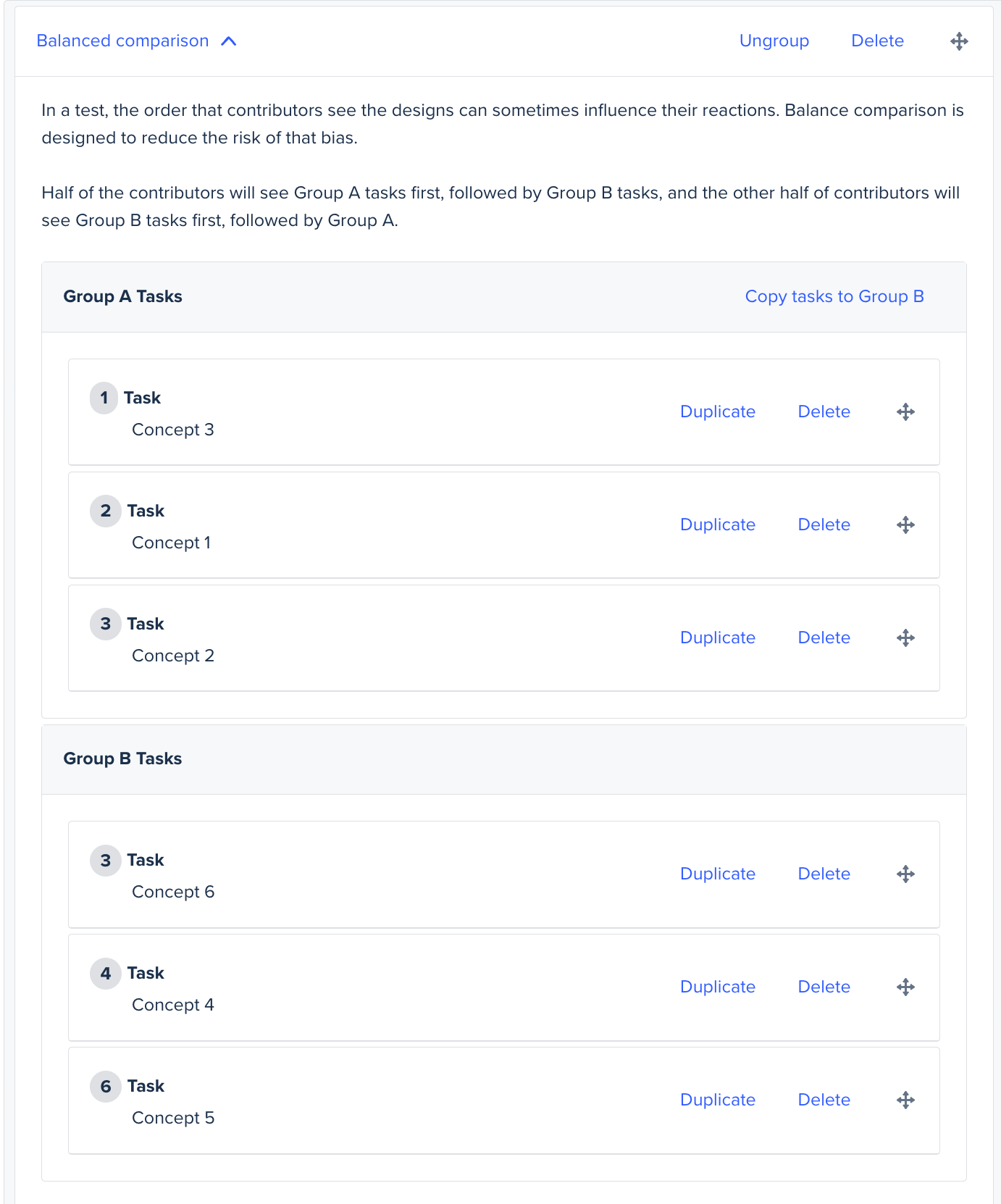Note: An equal number of contributors should take each study.

Explanation:
For 6 concepts (let's call them concept 1/2/3/4/5/6), three studies will need to be run. The first study will have concept 1 and 2 and 3 in Group A Tasks, and concept 4 and 5 and 6 in Group B Tasks.

The second study will have concept 2 and 3 and 1 in Group A Tasks and concept 5 and 6 and 4 in Group B Tasks.

The third study should have the concepts 3 and 1 and 2 in Group A Tasks and concepts 6 and 4 and 5 in Group B Tasks.

This is how contributors will be shown your concepts:

Study 1 - Half the contributors will see 1, 2, 3, 4, 5, 6, and the other half will see 4, 5, 6, 1, 2, 3.

Study 2 - Half the contributors will see 2, 3, 1, 5, 6, 4, and the other half will see 5, 6, 4, 2, 3, 1.

Study 3 - Half the contributors will see 3, 1, 2, 6, 4, 5, and the other half will see 6, 4, 5, 3, 1, 2.